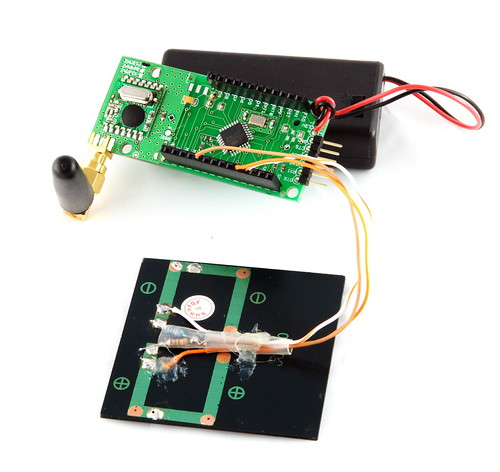# Solar Powered Widget

One of the tasks I want to use the widget boards for is a Wireless Sensor Network around the house for measuring environmental values. The sensors that I want to live outdoors will need to be self sufficient in terms of power, so I wanted to see if I could charge the batteries via , of course this can be done, but again I want to do it as cheaply and as simply as possible, I also want the widget board to monitor the voltage and report it back.

I already had a 3.6v 0.2w cell that I picked up some time ago from Sure Electronics for about \$10 for 5. The spec for this cell is max voltage (under load) is 3.6v and max current is 60mA, more than enough to trickle charge x2 AA NIMH batteries.

The Batteries should not require much of a top up as the node will be sleeping most of the time, from the power saving discussion @ JEELabs, by adding the solar, I should be able to just leave the node without having to change batteries (replacing failed batteries aside).

To measure the voltage produced by the solar cell I need a voltage divider that would produce 1.1v max @ 3.6v.

Why 1.1v I hear you ask? The reason for the A/D full scale input voltage of 1.1V is that I’ll be using the ATMega168 internal bandgap reference as the AREF source for the onboard A/D and not the default AREF.

There are a couple for reasons for this. The internal bandap reference itself is a 1.1v voltage reference, because the battery voltage may change over time (remember I’m not using any onboard voltage regulators) so as the VCC / battery voltage changes, so would my A/D voltage readings unless I use a reference voltage. Now as the reference is 1.1v this means that my input must not go above 1.1v else I will go over scale and get an incorrect reading.

I’m not going to bore you with the math so a quick calculation using an online voltage divider calculator (http://www.daycounter.com/Calculators/Voltage-Divider-Calculator.phtml)

Input Voltage = 3.6v

Output Voltage = 1.0v (remember I don’t want to go over 1.1V so I’ve given myself some headroom)

Gave the values for R1 = 26K and R2 = 10K

Luckily I had both these values, however for the 26K I only had one in 5% tolerance, the 10K I had as 1% (I like to use low tolerance resistors for voltage dividers so my error is minimised),upon testing the 26K resistor actually read 26.4K on my meter, so after plugging these real values in my actual FS Vout is 0.989v, pretty close to 1.0V.

A blocking diode is also required to prevent any damage to the Solar cell by the batteries, see the circuit and photo below.

(Click for larger image)(Click for larger image and notes)Sketch to read the voltage produced by the solar cell, next step is to find a waterproof housing and add some environmental sensors.

Another benefit of taking voltage readings of the solar cell is I now have a light sensor.

`int raw_solar = 0;        // Raw Solar FS ~ 1.0v`

`int raw_bandgap = 0;      // Intenal bandgap reference`

`float volt_solar = 0.0;`

` `

`void setup(){`

`  Serial.begin(57600);`

`  analogReference(INTERNAL);  // select internal reference for AREF`

`}`

` `

` `

`void loop(){`

`  `

`  raw_solar = analogRead(0);                         // read solar voltage raw values`

`  volt_solar = (raw_solar * 1.1 * 3.617) / 1024;     // Scale ADC input.  (voltage divider r1= 26.4k  r2 = 10k  vi=vo/(r2/(r1+r2)) = vo/.2777 =  vo * 3.617`

`  Serial.print(volt_solar);                          // display raw solar voltage reading`

`  Serial.println("v");  `

`  delay(1000);                    `

`  `

`}`

## 2 thoughts on “Solar Powered Widget”

This site uses Akismet to reduce spam. Learn how your comment data is processed.